## Optical instruments

8-5-99

### Making things look bigger

When you use an optical instrument, whether it be something very simple like a magnifying glass, or more complicated like a telescope or microscope, you're usually trying to make things look bigger so you can more easily see fine details. One thing to remember about this is that if you want to make things look bigger, you're always going to use converging mirrors or lenses. Diverging mirrors or lenses always give smaller images.

When using a converging lens, it's helpful to remember these rules of thumb. If the object is very far away, the image will be tiny and very close to the focal point. As the object moves towards the lens, the image moves out from the focal point, growing as it does so. The object and image are exactly the same size when the object is at 2F, twice the focal distance from the lens. Moving the object from 2F towards F, the image keeps moving out away from the lens, and growing, until it goes to infinity when the object is at F, the focal point. Moving the object still closer to the lens, the image steadily comes in towards the lens from minus infinity, and gets smaller the closer the object is to the lens.

Note that similar rules of thumb apply for a converging mirror, too.

### Multiple lenses

Many useful devices, such as microscopes and telescopes, use more than one lens to form images. To analyze any system with more than one lens, work in steps. Each lens takes an object and creates an image. The original object is the object for the first lens, and that creates an image. That image is the object for the second lens, and so on. We won't use more than two lenses, and we can do a couple of examples to see how you analyze problems like this.

### A microscope

A basic microscope is made up of two converging lenses. One reason for using two lenses rather than just one is that it's easier to get higher magnification. If you want an overall magnification of 35, for instance, you can use one lens to magnify by a factor of 5, and the second by a factor of 7. This is generally easier to do than to get magnification by a factor of 35 out of a single lens.

A microscope arrangement is shown below, along with the ray diagram showing how the first lens creates a real image. This image is the object for the second lens, and the image created by the second lens is the one you'd see when you looked through the microscope.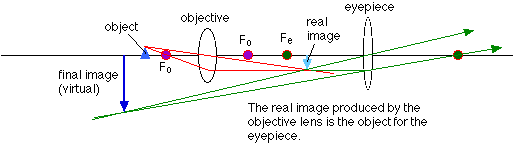Note that the final image is virtual, and is inverted compared to the original object. This is true for many types of microscopes and telescopes, that the image produced is inverted compared to the object.

### An example using the microscope

Let's use the ray diagram for the microscope and work out a numerical example. The parameters we need to specify are: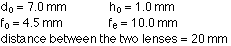To work out the image distance for the image formed by the objective lens, use the lens equation, rearranged to: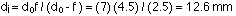The magnification of the image in the objective lens is: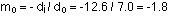So the height of the image is -1.8 x 1.0 = -1.8 mm.

This image is the object for the second lens, and the object distance has to be calculated:The image, virtual in this case, is located at a distance of:The magnification for the eyepiece is: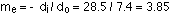So the height of the final image is -1.8 mm x 3.85 = -6.9 mm.

The overall magnification of the two lens system is: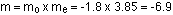This is equal to the final height divided by the height of the object, as it should be. Note that, applying the sign conventions, the final image is virtual, and inverted compared to the object. This is consistent with the ray diagram.

### Telescopes

A telescope needs at least two lenses. This is because you use a telescope to look at an object very far away, so the first lens creates a small image close to its focal point. The telescope is designed so the real, inverted image created by the first lens is just a little closer to the second lens than its focal length. As with the magnifying glass, this gives a magnified virtual image. This final image is also inverted compared to the original object. With astronomical telescopes, this doesn't really matter, but if you're looking at something on the Earth you generally want an upright image. This can be obtained with a third lens.

Note that the overall effect of the telescope is to magnify, which means the absolute value of the magnification must be larger than 1. The first lens (the objective) has a magnification smaller than one, so the second lens (the eyepiece) must magnify by a larger factor than the first lens reduces by. To a good approximation, the overall magnification is equal to the ratio of the focal lengths. With o standing for objective and e for eyepiece, the magnification is given by:

m = - fo / fe, with the minus sign meaning that the image is inverted.

### Resolving power

The resolving power of an optical instrument, such as your eye, or a telescope, is its ability to separate far-away objects that are close together into individual images, as opposed to a single merged image. If you look at two stars in the sky, for example, you can tell they are two stars if they're separated by a large enough angle. Some stars, however, are so close together that they look like one star. You can only see that they are two stars by looking at them through a telescope. So, why does the telescope resolve the stars into separate objects while your eye can not? It's all because of diffraction.

If you look at a far-away object, the image of the object will form a diffraction pattern on your retina. For two far-away objects separated by a small angle, the diffraction patterns will overlap. You are able to resolve the two objects as long as the central peaks in the two diffraction patterns don't overlap. The limit is when one central peak falls at the position of the first dark fringe for the second diffraction pattern. This is known as the Rayleigh criterion. Once the two central peaks start to overlap, in other words, the two objects look like one.

The size of the central peak in the diffraction pattern depends on the size of the aperture (the opening you look through). For your eye, this is your pupil. A telescope, or even a camera, has a much larger aperture, and therefore more resolving power. The minimum angular separation is given by: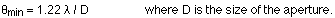The factor of 1.22 applies to circular apertures like your pupil, a telescope, or a camera lens.

The closer you are to two objects, the greater the angular separation between them. Up close, then, two objects are easily resolved. As you get further from the objects, however, they will eventually merge to become one.

### X-ray diffraction

Things that look a lot like diffraction gratings, orderly arrays of equally-spaced objects, are found in nature; these are crystals. Many solid materials (salt, diamond, graphite, metals, etc.) have a crystal structure, in which the atoms are arranged in a repeating, orderly, 3-dimensional pattern. This is a lot like a diffraction grating, only a three-dimensional grating. Atoms in a typical solid are separated by an angstrom or a few angstroms;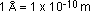. This is much smaller than the wavelength of visible light, but x-rays have wavelengths of about this size. X-rays interact with crystals, then, in a way very similar to the way light interacts with a grating.

X-ray diffraction is a very powerful tool used to study crystal structure. By examining the x-ray diffraction pattern, the type of crystal structure (i.e., the pattern in which the atoms are arranged) can be identified, and the spacing between atoms can be determined.

The two diagrams below can help to understand how x-ray diffraction works. Each represents atoms arranged in a particular crystal structure.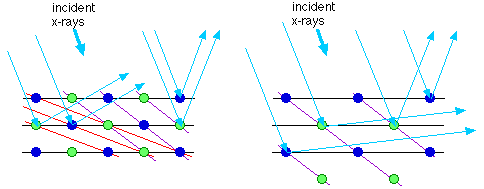You can think of the diffraction pattern like this. When x-rays come in at a particular angle, they reflect off the different planes of atoms as if they were plane mirrors. However, for a particular set of planes, the reflected waves interfere with each other. A reflected x-ray signal is only observed if the conditions are right for constructive interference. If d is the distance between planes, reflected x-rays are only observed under these conditions: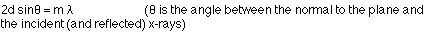That's known as Bragg's law. The important thing to notice is that the angles at which you see reflected x-rays are related to the spacing between planes of atoms. By measuring the angles at which you see reflected x-rays, you can deduce the spacing between planes and determine the structure of the crystal.